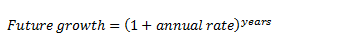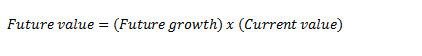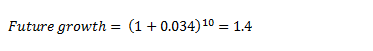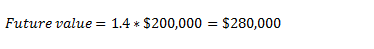# How to Calculate the Future Value of Real Estate Investments

Mar 13, 2016 by Millionacres Staff

There are a couple of major financial considerations when making a real estate investment. First, you want to estimate the property's potential to generate rental income, which can usually be done by evaluating the rental histories of similar properties nearby.

It can also be useful to estimate what the property might be worth in the future. For instance, if a rental property barely breaks even on rental income, but you project that you'll be able to sell it at a nice profit later; it still may be worth considering. With this in mind, here's how to calculate future value of real estate, and how to use this information.

Calculating the potential future value of real estate
First, you'll need to determine your projected growth rate. Real estate has historically appreciated at a rate of between 3% and 5% per year, depending on the price index you're looking at. The U.S. House Price Index shows that prices have risen at 3.4% per year on average since 1991, so we'll use that to illustrate our calculations. For the purposes of our calculation, you'll need to convert this to a decimal, or 0.034.

To calculate the expected future value based on your growth rate, add one to the rate, and raise this to a power equal to the number of years you're looking at. As a mathematical formula:Finally, multiply this future growth factor by the current value of the property.An example
For example, let's say that you buy an investment property worth \$200,000, and you'd like to estimate what its value will be in 10 years. Using our 3.4% average rate, we can calculate the future growth factor as follows:Multiplying this factor by the current value of \$200,000 gives us the potential future value of the property.Take this with a grain of salt
While historical averages for investment performance tend to be pretty reliable over long time periods, keep in mind that nobody has a crystal ball that can tell us an accurate projection of real estate values over the coming years. Sure, the long-term average is about 3.4%, but this includes the period from 2000 to 2006, when home prices grew more than 10% per year, and also includes the 2007 to 2012 period where prices dropped by more than 5% per year.

The point is that estimating the future value of a real estate investment can be a useful part of determining your profit potential, but by no means should you expect the property to be worth exactly this amount.

## 11% of the mega-wealthy swear by this investment…

The richest in the world have made their fortunes in many ways, but there is one common thread for many of them: They made real estate a core part of their investment strategy. Of all the ways the ultra-rich made their fortunes, real estate outpaced every other method 3 to 1.

If you, too, want to invest like the wealthiest in the world, we have a complete guide on what you need to take your first steps. Take the first step toward building real wealth by getting your free copy today. Simply click here to receive your free guide.